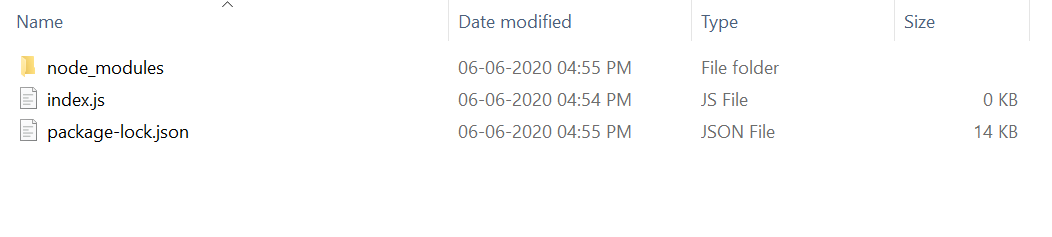# Moment.js moment().diff() Function

• Last Updated : 29 Jul, 2020

The moment().diff() function is used to get the difference in milliseconds of given dates which are passed as parameter to this function.

Syntax:

`moment().diff(Moment|String|Number|Date|Array, String, Boolean);`

Parameters: This function has two parameters, first one is the date of type Moment|String|Number|Date|Array and second parameter is an optional parameter of boolean type which is used to get floating numbers as result instead of integer.

Return Value: This function returns the date in milliseconds.

Installation of moment module:

1. You can visit the link to Install moment module. You can install this package by using this command.
`npm install moment`
2. After installing the moment module, you can check your moment version in command prompt using the command.
`npm version moment`
3. After that, you can just create a folder and add a file for example, index.js as shown below.

Example 1: Filename: index.js

 `// Requiring the module``const moment = require(``'moment'``);`` ` `var` `dateOne = moment([2019, 03, 17]);``var` `dateTwo = moment([2001, 10, 28]);`` ` `// Function call``var` `result = dateOne.diff(dateTwo, ``'days'``) `` ` `console.log(``"No of Days:"``, result)`

Steps to run the program:

1. The project structure will look like this:2. Run index.js file using below command:
`node index.js`

Output:

```No of Days: 6349
```

Example 2: Filename: index.js

 `// Requiring the module``const moment = require(``'moment'``);``  ` `function` `getYearDiff(dateOne, dateTwo) {``   ``return` `dateOne.diff(dateTwo, ``'years'``, ``true``);``}``  ` `// Function call``var` `result = getYearDiff(moment([2019, 11, ``    ``30]), moment([2001, 2, 17]));`` ` `console.log(``"No of years difference:"``, result)`

Steps to run the program:

1. The project structure will look like this:2. Run index.js file using below command:
`node index.js`

Output:

```No of years difference: 18.78611111111111
```

My Personal Notes arrow_drop_up